How Cheenta works to ensure student success?
Explore the Back-Story

# Test of Mathematics Solution Subjective 79 -Trigonometric InequalityThis is a Test of Mathematics Solution Subjective 79 (from ISI Entrance). The book, Test of Mathematics at 10+2 Level is Published by East West Press. This problem book is indispensable for the preparation of I.S.I. B.Stat and B.Math Entrance.

Also visit: I.S.I. & C.M.I. Entrance Course of Cheenta

## Problem

Let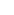,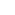, ... ,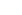be any values in the closed interval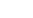. Show that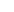=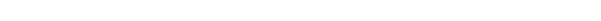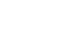.
What is the maximum value attainable byand at what values of,, ... ,, is the maximum value attained?

## Solution=Now we will show that for any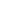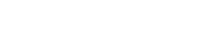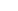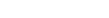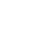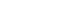Now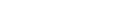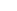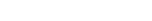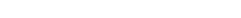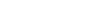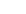[ as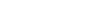]So for any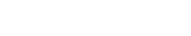So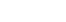(proved)
Maximum value attained byis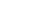and will be attained for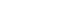for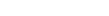This is a Test of Mathematics Solution Subjective 79 (from ISI Entrance). The book, Test of Mathematics at 10+2 Level is Published by East West Press. This problem book is indispensable for the preparation of I.S.I. B.Stat and B.Math Entrance.

Also visit: I.S.I. & C.M.I. Entrance Course of Cheenta

## Problem

Let,, ... ,be any values in the closed interval. Show that=.
What is the maximum value attainable byand at what values of,, ... ,, is the maximum value attained?

## Solution=Now we will show that for anyNow[ as]So for anySo(proved)
Maximum value attained byisand will be attained forfor### Knowledge Partner